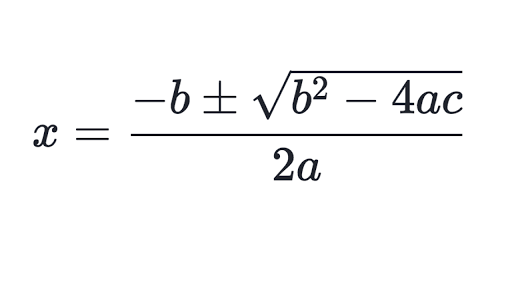# Polynomials

#### Information

In order to find the roots of a polynomial we first need to look at the quadratic formula (shown below) which allows us to quickly find the roots to a quadratic equation (a polynomial up to x2)In order to use the quadratic formula we first must know that:

a represents the coefficient of x2

b represents the coefficient of x

c represents the constant

e.g. in the polynomial 3x2 + 2x + 1

a = 3, b = 2, c = 1

#### Introducing Complex Numbers

A complex number is a number that has both a real and imaginary part

The real part are the numbers we normally see such as integers, fractions and roots

The imaginary part is where there is a √-1 also known as i (i = √-1)

An example of where you might see a complex number is the root of a polynomial such as

x2 - 4x + 5

Inputting a = 1, b = -4 and c = 5 into the quadratic formula gives us the roots:

x = 2 + i and 2 - i

This is called a complex conjugate (a pair of complex numbers), this will always be the case where if you have a root of 2 + i you will have the complex conjugate of 2 - i

This can also be written down as 2 ± i

(i = √-1, i2 = -1, i3 = -√-1 = -i)

*Important*

The complex conjugate of z is z*

where z represents a complex number

#### Finding Roots in Cubic Equations

When confronted by a cubic equation we are unable to use the quadratic equation as there are now 3 roots to the equation and not 2 roots

We instead have to employ a different strategy to find the roots shown easily in an example

If we have the cubic equation of:

x3 - x2 - 8x + 12

We first find the factors of the constant 12 which are:

±1, ±2, ±3, ±4, ±6, ±12

We then input these factors in the place of x until the equation = 0 such as:

(1)3 - (1)2 - 8(1) + 12 = 0

1 - 1 - 8 + 12 = 0

4 = 0 as this is not true (x-1) is not a factor so we move onto the next factor

(2)3 - (2)2 - 8(2) + 12 = 0

8 - 4 - 16 + 12 = 0 which is true so (x - 2) is a root

(If no positive factor leads to the equation being true switch to the negative factors)

We then need to divide the original equation by (x - 2)

This leaves us with the equation (x-2)(x2 + x - 6)

Using the quadratic formula to find the roots of x2 + x - 6 we find that:

(x - 2)(x - 2)(x + 3) = 0

Meaning the roots of the equation are:

x = 2, x = 2 and x = -3

As only when x is these values the equation is true